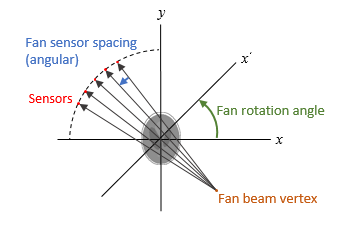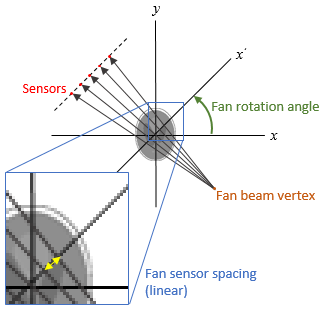# fan2para

Convert fan-beam projections to parallel-beam

## Syntax

P = fan2para(F,D)
P = fan2para(F,D,Name,Value)
[P,parallel_sensor_positions,parallel_rotation_angles] = fan2para(___)

## Description

P = fan2para(F,D) converts the fan-beam data F to the parallel-beam data P. Each column of F contains the fan-beam data at one rotation angle. D is the distance from the fan-beam vertex to the center of rotation.

example

P = fan2para(F,D,Name,Value) uses name-value pairs to control aspects of the data conversion.
[P,parallel_sensor_positions,parallel_rotation_angles] = fan2para(___) returns the parallel-beam sensor locations in parallel_sensor_positions and rotation angles in parallel_rotation_angles.

## Examples

collapse all

Create synthetic parallel-beam data.

ph = phantom(128);

Calculate the parallel beam transform and display it.

theta = 0:179; [Psynthetic,xp] = radon(ph,theta); imshow(Psynthetic,[],... 'XData',theta,'YData',xp,'InitialMagnification','fit') axis normal title('Synthetic Parallel-Beam Data') xlabel('\theta (degrees)') ylabel('x''') colormap(gca,hot), colorbarConvert the parallel-beam data to fan-beam.

Fsynthetic = para2fan(Psynthetic,100,'FanSensorSpacing',1);

Recover original parallel-beam data.

[Precovered,Ploc,Pangles] = fan2para(Fsynthetic,100,... 'FanSensorSpacing',1,... 'ParallelSensorSpacing',1); figure imshow(Precovered,[],... 'XData',Pangles,'YData',Ploc,'InitialMagnification','fit') axis normal title('Recovered Parallel-Beam Data') xlabel('Rotation Angles (degrees)') ylabel('Parallel Sensor Locations (pixels)') colormap(gca,hot), colorbar## Input Arguments

collapse all

Fan-beam projection data, specified as a numeric matrix. Each column of F contains the fan-beam data at one rotation angle. The number of columns indicates the number of fan-beam rotation angles and the number of rows indicates the number of fan-beam sensors.

Data Types: double | single

Distance in pixels from the fan beam vertex to the center of rotation, specified as a positive number. fan2para assumes that the center of rotation is the center point of the projections, which is defined as ceil(size(F,1)/2). The figure illustrates D in relation to the fan-beam vertex for one fan-beam projection.Data Types: double | single

### Name-Value Arguments

Specify optional comma-separated pairs of Name,Value arguments. Name is the argument name and Value is the corresponding value. Name must appear inside quotes. You can specify several name and value pair arguments in any order as Name1,Value1,...,NameN,ValueN.

Example: P = fan2para(F,D,'FanRotationIncrement',5)

Range of fan-beam rotation, specified as the comma-separated pair consisting of 'FanCoverage' and one of these values.

• 'cycle' — Rotate through the full range [0, 360) degrees.

• 'minimal' — Rotate through the minimum range necessary to represent the object.

Fan-beam rotation angle increment in degrees, specified as the comma-separated pair consisting of 'FanRotationIncrement' and a positive scalar.Data Types: double

Fan-beam sensor positioning, specified as the comma-separated pair consisting of 'FanSensorGeometry' and one of the following values.

Value

Meaning

Diagram

'arc'

Sensors are spaced at equal angles along a circular arc at distance D from the center of rotation.

FanSensorSpacing defines the angular spacing in degrees.'line'

Sensors are spaced at equal distances along a line that is parallel to the x' axis. The closest sensor is distance D from the center of rotation.

FanSensorSpacing defines the distance between fan-beams on the x' axis, in pixels.Fan-bean sensor spacing, specified as the comma-separated pair consisting of 'FanSensorSpacing' and a positive scalar.

• If FanSensorGeometry is 'arc', then FanSensorSpacing defines the angular spacing in degrees.

• If FanSensorGeometry is 'line', then FanSensorSpacing defines the linear distance between fan-beams, in pixels. Linear spacing is measured on the x' axis.

Data Types: double

Type of interpolation used between the parallel-beam and fan-beam data, specified as the comma-separated pair consisting of 'Interpolation' and one of these values.

'nearest' — Nearest-neighbor

'linear' — Linear (the default)

'spline' — Piecewise cubic spline

'pchip' — Piecewise cubic Hermite (PCHIP)

Range of parallel-beam rotation, specified as the comma-separated pair consisting of 'ParallelCoverage' and one of these values.

• 'cycle' — Parallel data covers the full range of [0, 360) degrees.

• 'halfcycle' — Parallel data covers [0, 180) degrees.

Parallel-beam rotation angle increment in degrees, specified as the comma-separated pair consisting of 'ParallelRotationIncrement' and a positive scalar k such that 180/k is an integer. If you do not specify ParallelRotationIncrement, then the default value is equal to FanRotationIncrement.

Data Types: double

Parallel-beam sensor spacing in pixels, specified as the comma-separated pair consisting of 'ParallelSensorSpacing' and a positive scalar. The range of parallel-beam sensor locations is computed from the range of fan angles, fanangles, according to: [D*sin(min(fanangles)) D*sin(max(fanangles))].

If you do not specify ParallelSensorSpacing, then the spacing is assumed to be uniform and is set to the minimum spacing implied by the fan angles and sampled over the range implied by the fan angles.

Data Types: double

## Output Arguments

collapse all

Parallel-beam projection data, returned as a numeric matrix. Each column of P contains the parallel-beam data at one rotation angle. The number of columns indicates the total number of parallel-beam rotation angles and is equal to the length of parallel_rotation_angles. The number of rows indicates the total number of parallel-beam sensors and is equal to the length of parallel_sensor_positions.

Data Types: double

Parallel-beam sensor locations, returned as a numeric column vector.

Data Types: double

Parallel-beam rotation angles, returned as a numeric row vector.

Data Types: double